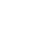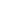——中国科学院办院方针

## 东北地理所在全国内陆沼泽湿地栖息地风险预测方面取得进展

2022-06-28 东北地理与农业生态研究所
【字体：湿地是地球上生产力最高的生态系统之一，为人类社会提供了一系列重要的生态服务功能。然而，当前湿地面临着来自气候变化和人类活动的双重胁迫。为了明确不同类型环境因子如何影响沼泽湿地物种的生境适宜性，并为气候变化下优先保护物种和区域的选取提供准确科学指导。中国科学院东北地理与农业生态研究所基于调查和标本数据，结合气候环境大数据，运用优化生态位模型，探究中国沼泽湿地植物物种生境适宜性关键驱动因子及敏感性，并对中国各区域沼泽湿地未来气候变化下的潜在风险进行评估，对优先保护物种和区域进行筛选，从而评估当前中国湿地保护工作在气候变化背景下的有效性及空缺，并提出可行的适应性保护策略。

研究表明：影响全国沼泽湿地植被物种生境适宜性的关键环境因子包括等温性、生长季节水热条件、最冷季度降水量等气候因子/土壤营养条件、pH等土壤因子，地下水位深度、地形坡度等地形水文因子/人类活动干扰程度；在未来气候变化的威胁下，中国本土湿地植物面临着适宜生境损失的风险，气候变化提高了非湿地物种和非本土物种的扩散和入侵能力；全国沼泽湿地的未来气候风险存在明显区域差异；大部分（>80%）预测的沼泽湿地气候变化热点区域未被纳入自然保护地体系中，完善沼泽湿地气候风险及湿地物种潜在响应的评估，并相应地设立新的湿地保护区，应是未来沼泽湿地适应性管理工作的重点。

近日，相关研究成果发表在Earth’s Future上。研究工作得到国家自然科学基金与吉林省的支持。图1.中国内陆沼泽湿地优势种发育关系及各环境因子重要性

表1 21世纪末RCP4.5/8.5情景下全国沼泽湿地植物优势种适宜区面积变化

 All (n=101) Wetland (n=87) Non-wetland (n=14) Woody (n=18) Non-woody (n=83) Net loss Net loss Net loss Net loss Net loss Net loss Net loss Net loss Net loss Net loss RCP 4.5 n=55 n=46 n=50 n=37 n=5 n=9 n=16 n=2 n=39 n=44 r=-32.33 r=39.15 r=-32.35 r=38.66 r=-32.11 r=42.29 r=-67.92 r=130.54 r=-28.25 r=38.94 a=-402.997 a=421.576 a=-371.690 a=360.127 a=-31.307 a=61.449 a=-87.219 a=3.317 a=-315.778 a=418.259 RCP 8.5 n=51 n=50 n=45 n=42 n=6 n=8 n=15 n=3 n=36 n=47 r=-37.11 r=38.72 r=-38.70 r=37.39 r=-24.24 r=50.76 r=-71.42 r=134.51 r=-32.80 r=38.50 a=-427.254 a=453.641 a=-396.539 a=394.720 a=-30.715 a=58.921 a=-91.647 a=3.543 a=-335.607 a=450.098

n: the number of species; r: the average change rate (%); a: the sum of changes in area(km2)© 1996 - 中国科学院 版权所有　京ICP备05002857号-1　京公网安备110402500047号　网站标识码bm48000002

© 1996 - 中国科学院 版权所有　京ICP备05002857号-1　京公网安备110402500047号　网站标识码bm48000002

© 1996 - 中国科学院 版权所有

86 10 68597289（总值班室）